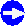# Gauss Law or Integral TheoremGauss law relates the charge contained inside a volume V bounded by a surface S to the flux of the electrical field.The flux of the electrical field through a surface S is the integral over the components of E perpendicular to the surface.The most simple way to visualize this is to equate the flux with the number of field lines running through the surface.The charge is usually expressed in terms of charge density r(x,y,z).Gauss law then states:
E ·   =   1    ·   r(x,y,z) · n · da e0 dV ó õ ó õ ó õ ó õ ó õ S VWith n = normal vector of the surface S, da = surface increment, dV = volume increment.Lets apply Gauss law to a capacitor with or without a dielectric inside. We have the following situation:Without a dielectric, all green field lines starting at the positve charges of the capacitor plates would run through the interior of the capacitor (and thus through the lower surface of the probing volume for applying Gauss' law).With a dielectric inside, only the "long" field lines from all field lines starting at the positive charges on the upper electrode will contribute to the flux of E because some of the green ones will end at the charges on the surface of the dielectric as shown in the enlargement of the probing volume.The number of green field lines ending at the surface charge of the dielectric is identical to the number of field lines that we would have inside the dielectric for the given polarization - where green and red ones meet, they cancel each other.We see that only the lower surface of our probing volume carries field lines, so the flux on this surface is number of field lines = field times one major area (= A) of the volume V.Without the dielectric, the flux would be larger because all flux lines starting at a positive charge would then contribute. The flux D in this simple case would be ,
D  = Eex · A
With A = that part of the surface S that contains field lines and Eex = field caused by the external charges only.With the dielectric, the flux is smaller as reasoned above. We conclude, using Gauss law, that the amount of charge inside the volume V must be reduced by the dielectric, which is quite obvious when we look at the picture.For a quantitative description lets compare the case with and without dielectric, realizing that the integrations called for in the formulations of Gauss law as given above are now simple multiplications:
Without dielectric With dielectric
Electrical flux with Gauss law
D0  =  E0 · A  = A · U
d
=  Q0
e0
Gauss law
Ddi  =  E · A   =  Q0 + Qpol
e0
Gauss law
with E0 = Field without the dielectric,
U = voltage applied to the capacitor,
d = distance between plates;
Q0 = charge on the plate within V,
A = area of the relevant side of V.
(Only one surface of V contributes)
with E = field inside the capacitor.

Qpol
A
is the polarization P by definition.
Electrical flux with Maxwell Definition
Rewriting the equations gives
Q0
A
=  e0 · E0   :=   D0
Maxwell
definition

Q0  +   Qpol
A
=   Ddi
=   Q0
A
+  P

=  D0 +  P

:=  e0·er · E
Maxwell definition
This is the definition of D, the electrical flux density This is the definition of er , the (relative) dielectric constant
Capacitance
The capacitance C is defined as C = Q/U = Q/E · d. Using the equations from above we have
C  =  Q0
E0 · d
=   A · e0 · E0
E0 · d
=   A · e0
d
C  =  Q
E · d
=   A · e0 · er · E
E · d
=   A · e0 · er
d
This is of course exactly what we would have expected
Linking the two systems
With P = e0 · c · E0 it follows

Ddi  =  e0 · E0 + e0 · c · E0
=  e0 · (1 + c) · E0
:=  e0 · er · E0

er  :=  1 + c
We thus obtain two simple equations connecting the old-fashioned "D and er" world with the modern "P and c" world

Ddi  =  D0 + P

er  =  1 + cOK - so it is tedious and boring. And the result is simple and we all know it.Still, try at least once in your life to understand completely the reasoning behind this. Similar stuff will come up all the time!3.1.1 Dielectrics5.2.1 Interaction between Light and MatterSolution to 3.2-1

© H. Föll (Advanced Materials B, part 1 - script)